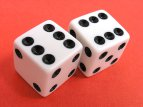### Probability of a 12 when you roll two DicePerforming probability questions in kdb/q is simple. I recently got asked how to find the probability of rolling a sum of 12 with two dice. We’ll look at two approaches to finding the likely outcomes in kdb/q:

### Method 1 – Enumeration of all possibilities

Step by step we:

1. Generate the possible outcomes for one die.
2. Generate all permutations for possible outcomes of two dice, find the sum of the dice.
3. Count the number of times each sum occurs and divide by all possible outcomes to get each probability.

### Method 2 – Monte Carlo Simulation

Alternatively , if it hadn’t occurred to us to use cross to generate all possible outcomes, or for situations where there may be too many to consider. We can use Monte Carlo method to simulate random outcomes and then similarly group and count their probability.

``` q)1+900000?/:6 6 / random pairs of dice rolls 2 5 6 6 2 4 3 1 5 1 1 4 6 5 3 4 2 4 1 2 3 6 6 1 1 3 6 3 6 2 3 1 5 4 4 4 6 3 1 3 1 2 5.. 4 4 4 5 1 4 3 6 5 1 4 4 3 4 1 1 1 5 4 5 2 4 4 4 5 2 1 2 6 5 2 4 3 1 4 2 1 1 3 4 6 5 1.. q)/ same as before, count frequency of each result q)(2+til 11)#{x%sum x} count each group sum each flip 1+900000?/:6 6 2 | 0.02766 3 | 0.05559 4 | 0.08347 5 | 0.11085 6 | 0.1391822 7 | 0.1669078 8 | 0.1386156 9 | 0.1112589 10| 0.08317222 11| 0.05556222 12| 0.02773111 ```

Therefore the probability of rolling a sum of 12 with two dice is 1/36 or 0.27777. Here’s the similar dice permutation problem performed in java.

### Kdb Problem Questions

If you want to try using the monte carlo method yourself try answering these questions:

1. The Birthday Paradox: How many people do you need before the odds are good (greater than 50%) that at least two of them share a birthday?
2. Finding the value of Pi. Consider a square at the origin of a coordinate system with a side of length 1. Now consider a quarter circle inside of the square, this circle has a radius of 1, therefore its area is pi/4. For a point (X,Y) to be inside of a circle of radius 1, its distance from the origin (X ², Y²) will be less than or equal to 1. We can generate random (X,Y) positions and determine whether each of them are inside of the circle. The ratio of those inside to outside will give the area. (bonus points for using multiple threads)